# RS Aggarwal Class 10 Solutions Chapter 11 Arithmetic Progressions Ex 11D

## RS Aggarwal Class 10 Solutions Chapter 11 Arithmetic Progressions Ex 11D

These Solutions are part of RS Aggarwal Solutions Class 10. Here we have given RS Aggarwal Solutions Class 10 Chapter 11 Arithmetic Progressions Ex 11D

Other Exercises

Question 1.
Solution:
(3y – 1), (3y + 5) and (5y+ 1) are in AP
(3y + 5) – (3y – 1) = (5y + 1) – (3y + 5)
⇒ 2 (3y + 5) = (5y + 1) + (3y – 1)
⇒ 6y + 10 = 8y
⇒ 8y – 6y = 10
⇒ 2y = 10
⇒ y = 5
y = 5

Question 2.
Solution:
k, (2k – 1) and (2k + 1) are the three successive terms of an AP.
(2k – 1) – k = (2k + 1) – (2k – 1)
⇒ 2 (2k – 1) = 2k + 1 + k
⇒ 4k – 2 = 3k + 1
⇒ 4k – 3k = 1 + 2
⇒ k = 3
k = 3

Question 3.
Solution:
18, a, (b – 3) are in AP
⇒ a – 18 = b – 3 – a
⇒ a + a – b = -3 + 18
⇒ 2a – b = 15

Question 4.
Solution:
a, 9, b, 25 are in AP.
9 – a = b – 9 = 25 – b
b – 9 = 25 – b
⇒ b + b = 22 + 9 = 34
⇒ 2b = 34
⇒ b= 17
a – b = a – 9
⇒ 9 + 9 = a + b
⇒ a + b = 18
⇒ a + 17 = 18
⇒ a = 18 – 17 = 1
a = 18, b= 17

Question 5.
Solution:
(2n – 1), (3n + 2) and (6n – 1) are in AP
(3n + 2) – (2n – 1) = (6n – 1) – (3n + 2)
⇒ (3n + 2) + (3n + 2) = 6n – 1 + 2n – 1
6n + 4 = 8n – 2
⇒ 8n – 6n = 4 + 2
⇒ 2n = 6
⇒ n = 3
and numbers are
2 x 3 – 1 = 5
3 x 3 + 2 = 11
6 x 3 – 1 = 17
i.e. (5, 11, 17) are required numbers.

Question 6.
Solution:
Three digit numbers are 100 to 990 and numbers which are divisible by 7 will be
105, 112, 119, 126, …, 994
Here, a = 105, d= 7, l = 994
Tn = (l) = a + (n – 1) d
⇒ 994 = 105 + (n – 1) x 7
⇒ 994 – 105 = (n – 1) 7
⇒ (n – 1) x 7 = 889
⇒ n – 1 = 127
⇒ n = 127 + 1 = 128
Required numbers are 128

Question 7.
Solution:
Three digit numbers are 100 to 999
and numbers which are divisible by 9 will be
108, 117, 126, 135, …, 999
Here, a = 108, d= 9, l = 999
T(l) = a + (n – 1) d
⇒ 999 = 108 + (n – 1) x 9
⇒ (n – 1) x 9 = 999 – 108 = 891
⇒ n – 1 = 99
⇒ n = 99 + 1 = 100

Question 8.
Solution:
Sum of first m terms of an AP = 2m² + 3m
Sm = 2m² + 3m
S1 = 2(1)² + 3 x 1 = 2 + 3 = 5
S2 = 2(2)² + 3 x 2 = 8 + 6=14
S3 = 2(3)² + 3 x 3 = 18 + 9 = 27
Now, T2 = S2 – S1 = 14 – 5 = 9
Second term = 9

Question 9.
Solution:
AP is a, 3a, 5a, …
Here, a = a, d = 2a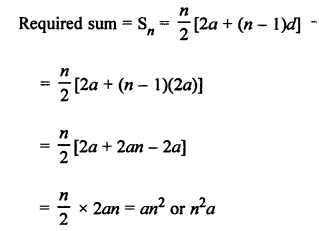Question 10.
Solution:
AP 2, 7, 12, 17, …… 47
Here, a = 2, d = 7 – 2 = 5, l = 47
nth term from the end = l – (n – 1 )d
5th term from the end = 47 – (5 – 1) x 5 = 47 – 4 x 5 = 47 – 20 = 27

Question 11.
Solution:
AP is 2, 7, 12, 17, …
Here, a = 2, d = 7 – 2 = 5
an = a + (n – 1) d = 2 + (n – 1) x 5 = 2 + 5n – 5 = 5n – 3
Now, a30 = 2 + (30 – 1) x 5 = 2 + 29 x 5 = 2 + 145 = 147
and a20 = 2 + (20 – 1) x 5 = 2 + 19 x 5 = 2 + 95 = 97
a30 – a20 = 147 – 97 = 50

Question 12.
Solution:
Tn = 3n + 5
Tn-1 = 3 (n – 1) + 5 = 3n – 3 + 5 = 3n + 2
d = Tn – Tn-1 = (3n + 5) – (3n + 2) = 3n + 5 – 3n – 2 = 3
Common difference = 3

Question 13.
Solution:
T= 7 – 4n
Tn-1 = 7 – 4(n – 1) = 7 – 4n + 4 = 11 – 4n
d = Tn – Tn-1 = (7 – 4n) – (11 – 4n) = 7 – 4n – 11 + 4n = -4
d = -4

Question 14.
Solution:
AP is √8, √18, √32, …..
⇒ √(4 x 2) , √(9 x 2) , √(16 x 2), ………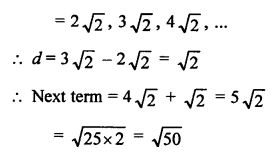Question 15.
Solution: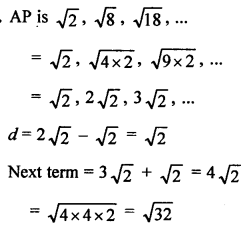Question 16.
Solution:
AP is 21, 18, 15, …n
Here, a = 21, d = 18 – 21 = -3, l = 0
Tn (l) = a + (n – 1) d
0 = 21 + (n – 1) x (-3)
0 = 21 – 3n + 3
⇒ 24 – 3n = 0
⇒ 3n = 24
⇒ n = 8 .
0 is the 8th term.

Question 17.
Solution:
First n natural numbers are 1, 2, 3, 4, 5, …, n
Here, a = 1, d = 1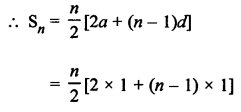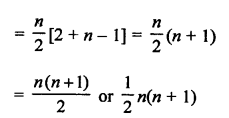Question 18.
Solution:
First n even natural numbers are 2, 4, 6, 8, 10, … n
Here, a = 2, d = 4 – 2 = 2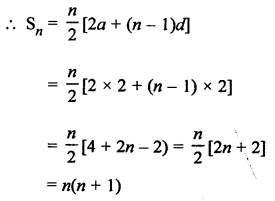Question 19.
Solution:
In an AP
First term (a) = p
and common difference (d) = q
T10 = a + (n – 1) d = p + (10 – 1) x q = (p + 9q)

Question 20.
Solution: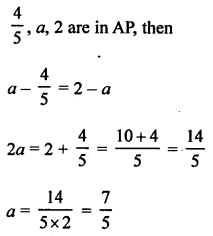Question 21.
Solution:
2p + 1, 13, 5p – 3 are in AP, then
13 – (2p + 1) = (5p – 3) – 13
⇒ 13 – 2p – 1 = 5p – 3 – 13
⇒ 12 – 2p = 5p – 16
⇒ 5p + 2p = 12 + 16
⇒ 7p = 28
⇒ p = 4
P = 4

Question 22.
Solution:
(2p – 1), 7, 3p are in AP, then
⇒ 7 – (2p – 1) = 3p – 7
⇒ 7 – 2p + 1 = 3p – 7
⇒ 7 + 1 + 7 = 3p + 2p
⇒ 5p = 15
⇒ p = 3
P = 3

Question 23.
Solution: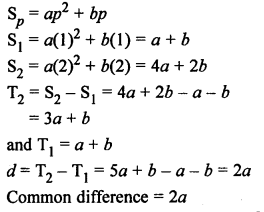Question 24.
Solution: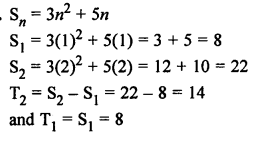d = T2 – T1 = 14 – 8 = 6
Common difference = 6

Question 25.
Solution: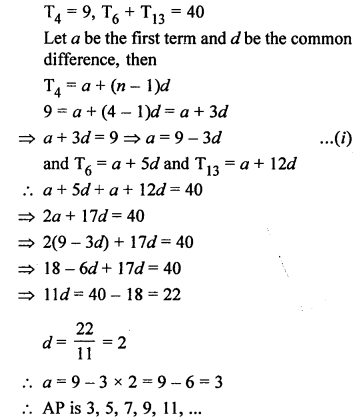Question 26.
Solution: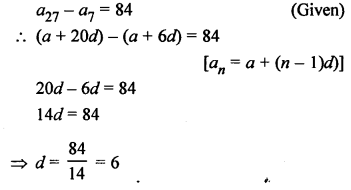Question 27.
Solution: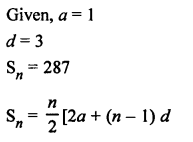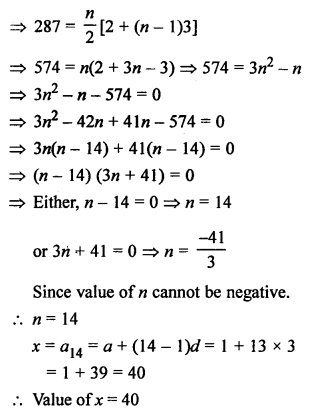Hope given RS Aggarwal Solutions Class 10 Chapter 11 Arithmetic Progressions Ex 11D are helpful to complete your math homework.

If you have any doubts, please comment below. Learn Insta try to provide online math tutoring for you.